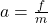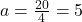## An unbalanced force of 20 N is applied to a 4.0 kg mass at rest. What is the acceleration of the object?

Question

An unbalanced force of 20 N is applied to a 4.0 kg mass at rest. What is the
acceleration of the object?

in progress 0
6 months 2021-08-13T01:38:01+00:00 1 Answers 2 views 0

## 5 m/s²

Explanation:

The acceleration of an object given it’s mass and the force acting on it can be found by using the formulaf is the force

m is the mass

We haveWe have the final answer as

### 5 m/s²

Hope this helps you